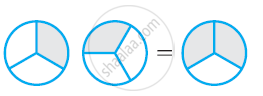Share

# Some Pictures (A) to (C) Are Given Below. Tell Which of Them Show: 2 Multiply 1by3 = 2by3 - CBSE Class 7 - Mathematics

ConceptMultiplication of Fractions Multiplication of a Fraction by a Whole Number

#### Question

Some pictures (a) to (c) are given below. Tell which of them show:

2 xx 1/3 = 2/3

a)b)c)#### Solution

2 xx 1/3 = 2/3  represents the addition of 2 figures, each representing 1 shaded part out of 3 equal parts and 2/3 represents 2 shaded parts out of 3 equal parts. Hence,2 xx 1/3 =  2/3 is represented by (a).

Is there an error in this question or solution?

#### APPEARS IN

NCERT Solution for Mathematics for Class 7 (2018 to Current)
Chapter 2: Fractions and Decimals
Ex. 2.20 | Q: 2.2 | Page no. 36
Solution Some Pictures (A) to (C) Are Given Below. Tell Which of Them Show: 2 Multiply 1by3 = 2by3 Concept: Multiplication of Fractions - Multiplication of a Fraction by a Whole Number.
S